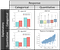# Measuring Correlation — simple techniques for categorical, discrete, and continuous data

A 5 minute, formula-free refresher on your favorite undervalued tools for data analysis!

Here are the four main ways to measure correlation, depending on what types of variables you have.Choosing the right tool, inspired by a similar grid from the Data Analysis Tools course

This boils down to just three main tests, so the trick is framing both your question and data in a workable format for either:

• Chi-squared test for independence — Compare proportions in groups
• Analysis of variance (ANOVA) — Analyze differences in group means
• Pearson’s correlation coefficient — Check for a linear relationship

We see that the chi-squared test is suitable whenever both of you explanatory and response variables are categorical, since we are comparing the frequency of some distinct response occurring in each group. Chi-squared is also (usually) suitable with a quantitative explanatory variable, once it is discretized into appropriate categories for your analysis. ANOVA is useful when comparing the average of a quantitative variable across different group categories. Lastly, Pearson’s correlation coefficient quantifies linear relationships between two quantitative variables.

The above chart is an adapted version from the Data Analysis Tools class on Coursera. The course is highly recommended for anyone already familiar with the above techniques but just needs a refresher in a more practical setting than a college textbook.

Assuming we live in the world of the null hypothesis, a p-value is the probability of the results from your sample being a random coincidence. It’s the chances of you observing another sample at least as extreme as the one you have. You want this p-value to be as low as possible when rejecting your null hypothesis, because it indicates that your result is the effect of some underlying relationship and not due to random noise. P-values can also be viewed as the type-I error rate. You can learn more about p-values in this blog post!

The dark horse of statistical tests. The whole “test for independence” comes from the general question of:

“Is a categorical response dependent on a categorical explanatory variable”

More pragmatically, we can compare the proportions of some response variable between two explanatory groups. The following hypothesis is typical for a chi-squared test, where the mean represents the percentage of outcomes in each sample.

Hₒ: μ₁ = μ₂

Hₐ: μ₁ μ₂

Chi-squared is really great because you can use it for almost any application by reframing the question and data. For example, everyone claims that each summer is hotter than the last. We can easily test this using chi-squared! Just transform the quantitative temperature response variable to categorical by either counting the percentage of record-breaking days or simply the number of days above x degrees.

Even if your explanatory variable is quantitative, you can always categorize that into whichever buckets suit your experiment. Do millennials really leave higher restaurant tips? Just compare the proportion of tips exceeding x% for the millennial age group against all others.

A test between three groups will have the following null and alternative hypothesis.

Hₒ: μ₁ = μ₂ = μ₃

Hₐ: μ₁ μ₂ μ₃

It’s important to emphasize that, in the alternative hypothesis, only one of the inequalities must be met to satisfy the statement. It does not require all means to be different. Or, as Ernie eloquently explains…

“one of these [means] is not like the other”.

As the name suggests, ANOVA is entirely based on variance even though it checks for differences in means across groups. It determines if group means really are different by testing the ratio of two variance measures:

1. Variance among the means of each group

2. Total variance within each group

One of the clearest examples that demonstrates why variance is so important is directly from the course material.

In country one, the means could be different but each group’s wide dispersion makes it questionable. Country two, however, has visibly different means among the three groups because of both the high variance between means and low variance within each group.

Lastly, we have the infamous Pearson’s correlation coefficient. The main point to keep in mind is that the correlation coefficient only indicates strength of linear relationships. The correlation coefficient might not recognize what otherwise could be considered a very strong relationship, such as below.

For reasons like this, it is always important to view the whole scatter plot before jumping to correlation coefficients. The other familiar term is , which is the fraction of response variability that can be explained by the explanatory variable. In other words, our explanatory variable can predict r²% of the variability in the response variable.

At this point you might be wondering:

“But what if I want to run Chi-squared tests across multiple categories?”

“How do I know which group differs in an ANOVA test?”

“What if my statistical relationship changes significantly when a 2nd explanatory variable is introduced?”

These important topics are beyond the scope of this 5-minute article, but the Coursera content covers such concerns regarding post-hoc tests and statistical interactions. Other courses in the specialization touch upon logistic regression, multiple regression, confidence intervals, and much more!

## Ro Data Team Blog

#### Ro Data Team Blog: data analytics, data engineering, data science